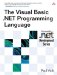# Operator Resolution

 <  Day Day Up  >

With the exception of the comparison operators, the result type of an operation is the same as the type of the operation's operands. In other words, adding two Short values together will result in a Short value. If that expression happens to be 32767S + 32767S , the operation will result in an overflow, even though the value 65534 could fit into a wider type, like Integer .

When a binary operation involves two types that are not the same ”for example, adding a Long and a Short together ”the result type of the operation will be the wider of the two types ”in this case, Long . This is because it is likely that the result of the operation will be a value that only fits into the wider of the two types. If the operator is being applied to a type for which it is not defined ”for example, Not 10.5 ”then the result of the operation will be the type that is closest to the operand type and is defined for the operator ”in this case, Long .

The most common example of this rule is the division operator ( / ), which is only defined for the types Single and Double . Dividing two integers using the ( / ) operator will always result in a Double , even if the result is an integer value.

Because String is neither wider nor narrower than the numeric types, it is treated specially. The result type of numeric operations that include String is always the numeric type, not String . So the operation 1 + "2" results in 3 , not the string "12" .

## Compatibility

In previous versions, operations on String and the numeric types resulted in a String value, the exact opposite of what happens in Visual Basic .NET. The behavior was changed because many programmers did not expect 1 + "2" to result in "12" .

When one of an operation's operands is typed as Object , the result type of the operation will be determined at runtime based on the types of the values and the value that results from the operation. Unlike operations on types other than Object , the result type of the operation will be widened if the result does not fit into the type of the operands.

` Dim s1, s2, s3 As Short Dim o As Object s1 = 32767 s2 = 32767 s3 = s1 + s2                ' Overflow o = CObj(s1) + CObj(s2)     ' Results in 65534 `

In this example, the first addition statement will result in an overflow because the result will not fit into a Short variable. However, the second statement at runtime will result in the Integer value 65534 .

 <  Day Day Up  >The Visual Basic .NET Programming Language
ISBN: 0321169514
EAN: 2147483647
Year: 2004
Pages: 173
Authors: Paul Vick

Similar book on Amazon